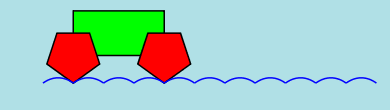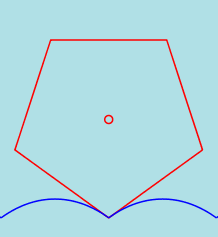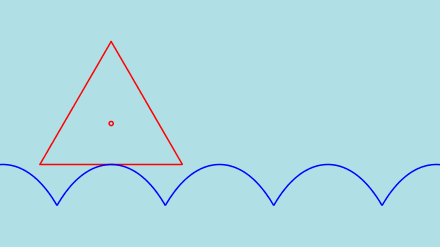Polygonal wheelsDid you ever see a car with square wheels that nevertheless rolls smoothly along a rather strange road? We want to know more about the shape of this road.

A rolling straight line

We start with a horizontal straight line segment through the point A that we suppose as attached to the point O. We want to determine the shape of the road in such a way that the point O moves on a horizontal line as the line rolls over the road (without any slipping).Choosing the coordinate system as indicated on the drawing, the equation of the line in the start position is y = -1 and the origin moves along the x-axis as the line is rolling.

To obtain the equation y = f(x) of the road we express that the length of the curve AB is the same as the length of the line segment A'B. This yields a differential equation together with the initial condition f(0) = -1.

The solution of this initial value problem is y = - cosh(x). The function cosh is the "hyperbolic cosine function" that is linked to the exponential function by the relation cosh(x) = (exp(x)+exp(-x))/2. The curve represented by the equation y = cosh(x) is a "catenary", so the "road" we are looking for is an inverted catenary.

Square wheelsNow we consider a square wheel. It's easy to see that the appropriate road is a sequence of pieces of inverted catenaries. In the junction points the tangents to both parts of the road must correspond to the angle of the square as indicated on the figure.

Other polygonal wheels

It's rather easy to consider other wheels that have the form of a regular polygon. In every case the road consists of a sequence of pieces of inverted catenaries. The angle of the tangents in the cusps must correspond to the angle of the polygon.

We consider a car with wheels that have the shape of a regular PENTAGON.However... an equitriangular wheel will CRASH as can be seen in the following figures. A vertex of the rolling triangle hits the road before the vertex reaches the cusp.An interesting (and more general) paper about this subject is "Roads and Wheels" by Leon Hall and Stan Wagon and published in "Mathematics Magazine", Vol. 65, no 5, december 1992.

Last update: January 26, 2018.